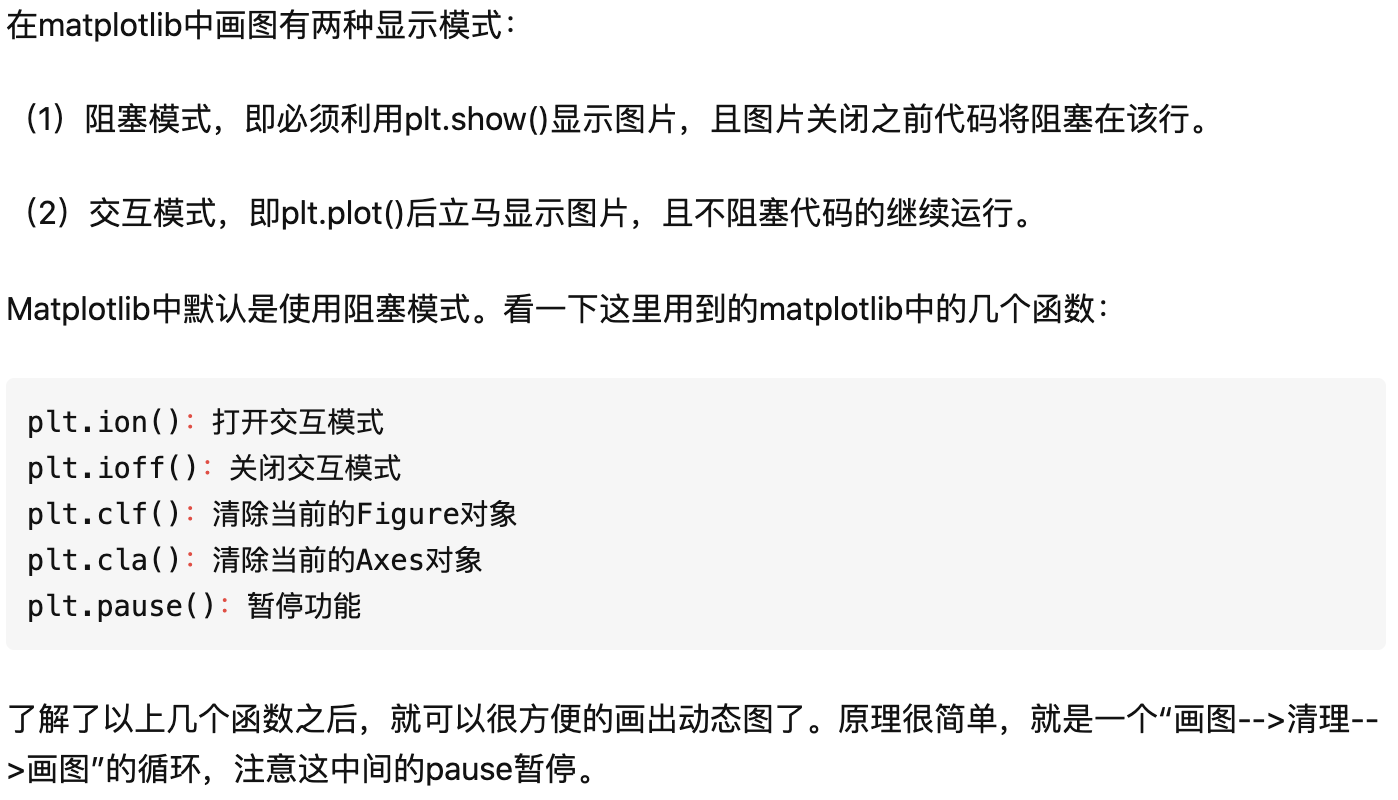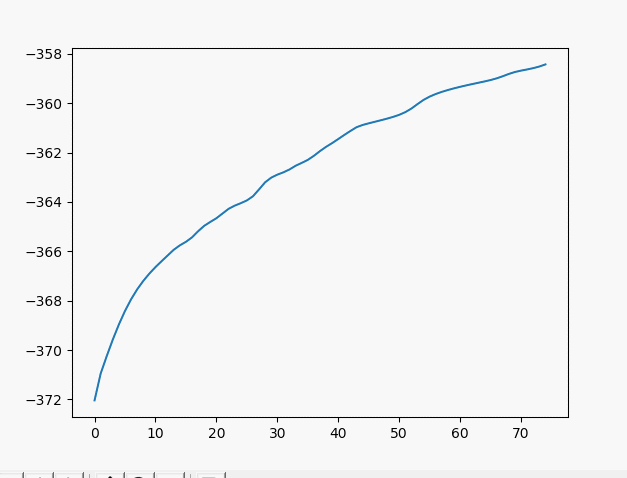• 使用plt.ion()这个函数，使matplotlib的显示模式转换为交互（interactive）模式。即使在脚本中遇到plt.show()，代码还是会继续执行。 import matplotlib.pyplot as plt plt.ion() # 打开交互模式 # 同时打开两个...
因为python可视化库matplotlib的显示模式默认为阻塞（block）模式（即：在plt.show()之后，程序会暂停到那儿，并不会继续执行下去）。如何 展示动态图或多个窗口 呢？
使用plt.ion()这个函数，使matplotlib的显示模式转换为交互（interactive）模式。即使在脚本中遇到plt.show()，代码还是会继续执行。
import matplotlib.pyplot as plt
plt.ion()    # 打开交互模式
# 同时打开两个窗口显示图片
plt.figure()  #图片一
plt.imshow(i1)
plt.figure()    #图片二
plt.imshow(i2)
# 显示前关掉交互模式
plt.ioff()
plt.show()

在plt.show()之前一定不要忘了加plt.ioff()，如果不加，界面会一闪而过，并不会停留。
在交互模式下：
1、plt.plot(x)或plt.imshow(x)是直接出图像，不需要plt.show()。
2、如果在脚本中使用ion()命令开启了交互模式，没有使用ioff()关闭的话，则图像会一闪而过，并不会常留。要想防止这种情况，需要在plt.show()之前加上ioff()命令。
在阻塞模式下：
1、打开一个窗口以后必须关掉才能打开下一个新的窗口。这种情况下，默认是不能像Matlab一样同时开很多窗口进行对比的。
2、plt.plot(x)或plt.imshow(x)是直接出图像，需要plt.show()后才能显示图像。
参考链接：matplotlib 画动态图以及plt.ion()和plt.ioff()的使用
实战案例：PyTorch实战示例01——regression
展开全文matplotlib python
• plt.ion()：将 figure 设置为交互模式，figure 不用 plt.show() 也可以显示。 plt.ioff()：将 figure 设置为阻塞模式，也是 figure 的默认模式，figure 必须用 plt.show() 才能显示。 plt.show()：显示所有的 ...
 plt.ion()：将 figure 设置为交互模式，figure 不用 plt.show() 也可以显示。  plt.ioff()：将 figure 设置为阻塞模式，也是 figure 的默认模式，figure 必须用 plt.show() 才能显示。  plt.show()：显示所有的 figure（不管是阻塞模式的还是交互模式的）。若一个 figure 下一个 plt.show()，则只有关闭一个 figure，才会出现下一个 figure。若最后设置 plt.show()，则会显示设置的所有 figure。  运用代码加深理解： 4.1 交互模式下的尝试 import matplotlib.pyplot as plt
plt.ion()
plt.figure()
 4.2 阻塞模式下的尝试 import matplotlib.pyplot as plt
# plt.ioff()
plt.figure()
 4.3.1 混合模式下1 import matplotlib.pyplot as plt
plt.figure()
plt.ion()
plt.figure()
 4.3.2 混合模式下2 import matplotlib.pyplot as plt
plt.figure()
plt.ion()
plt.figure()
plt.show()
 4.4 show下的模式 import matplotlib.pyplot as plt
plt.figure()
plt.show()
plt.figure()
plt.show()
 
展开全文python 数据可视化 matplotlib
• 在训练神经网络时，我们常常希望在图中看到loss减小的动态过程，这时我们可用plt.ion()函数打开交互式模式，在交互式模式下可动态地展示图像。 PS:在plt.show()之前一定不要忘了加plt.ioff()，如果不加，界面会一闪...
前言
在训练神经网络时，我们常常希望在图中看到loss减小的动态过程，这时我们可用plt.ion()函数打开交互式模式，在交互式模式下可动态地展示图像。PS:在plt.show()之前一定不要忘了加plt.ioff()，如果不加，界面会一闪而过，并不会停留。
动态画图
import matplotlib.pyplot as plt
x = list(range(1, 21))  # epoch array
loss = [2 / (i**2) for i in x]  # loss values array
plt.ion()
for i in range(1, len(x)):
ix = x[:i]
iy = loss[:i]
plt.cla()
plt.title("loss")
plt.plot(ix, iy)
plt.xlabel("epoch")
plt.ylabel("loss")
plt.pause(0.5)
plt.ioff()
plt.show()

动态地展示多张图片
    f, a = plt.subplots(1, 1, figsize=(5, 5))
plt.ion()
for imgPath in imglist:
a.imshow(img); a.set_xticks(()); a.set_yticks(())
plt.pause(0.5)

plt.ioff()
plt.show()展开全文• plt.ion() # 创建循环 for i in range(100): x.append(i) # 添加i到x轴的数据中 y.append(i**2) # 添加i的平方到y轴的数据中 plt.clf() # 清除之前画的图 plt.plot(x, y * np.array([-1])) # 画出当前x列表和y...
from matplotlib import pyplot as plt
import numpy as np

# 创建实时绘制横纵轴变量
x = []
y = []

# 创建绘制实时损失的动态窗口
plt.ion()

# 创建循环
for i in range(100):
x.append(i)	# 添加i到x轴的数据中
y.append(i**2)	# 添加i的平方到y轴的数据中
plt.clf()  # 清除之前画的图
plt.plot(x, y * np.array([-1]))  # 画出当前x列表和y列表中的值的图形
plt.pause(0.001)  # 暂停一段时间，不然画的太快会卡住显示不出来
plt.ioff()  # 关闭画图窗口

下面以绘制神经网络损失值来展示效果：参考文章1：Python中matplotlib实时画图
参考文章2：利用matplotlib绘制多个实时刷新的动态图表
展开全文• 在使用matplotlib的过程中，常常会需要画很多图，但是好像并不能同时...这就要使用plt.ion()这个函数，使matplotlib的显示模式转换为交互（interactive）模式。即使在脚本中遇到plt.show()，代码还是会继续执行。下面这机器学习
• Python学习|matplotlib的plt.ion()图像闪退 plt.ion()和plt.ioff()需要一起出现不然闪退
• 在使用matplotlib的过程中，常常会需要画很多图，但是好像并不能同时展示许多图。这是因为python可视化库matplotlib的显示模式默认为阻塞（block）模式。什么是阻塞模式那？...这就要使用plt.ion()这个函数，使m...
• 只将建立神经网络定义了类——class Net(torch.nn.Module)，我把训练过程、参数回传、优化器选择以及可视化分别做了函数），供以后直接调用，但是当做到可视化的时候，就遇到了问题，而问题就出在plt.ion()和plt....python matplotlib 可视化 神经网络
• 这个链接的方法反复试过了，plt.ioff()就是无法达到图片显示的效果，后来发现只要输入plt.ion() 就不能显示图片, 输入plt.ioff() 关闭交互模式就可以显示图片了，但是程序恢复阻塞。 ...
• 代码如下： import matplotlib.pyplot as plt x = list(range(1, 100)) # epoch array loss = [10 / (i**2) for i in x] # loss values array ...plt.ion() for i in range(1, len(x)): ix = x[:i] iy = loss[:...
• import matplotlib.pyplot as plt x = list(range(1, 100)) # epoch array loss = [10 / (i**2) for i in x] # loss values array plt.ion() for i...
• import matplotlib.pyplot as plt x = list(range(1, 100)) # epoch array loss = [10 / (i**2) for i in x] # loss values array ...plt.ion() for i in range(1, len(x)): ix = x[:i] iy = loss...
• 在用matplotlib时，plt.ion()的动态显示始终显示不出来。 经测试。Pycharm需要让plot视图单独弹出视窗才可以动态显示。 File->Settings->Tools->Python scientific->取消Show plots in tool window前...Python
• 在学习Tensorflow过程中，总是无法动态显示红色拟合曲线。测试代码如下（莫烦大佬）： # coding:UTF-8 ...import matplotlib.pyplot as plt os.environ['TF_CPP_MIN_LOG_LEVEL'] = '2' # 忽略不必要的警告 ...python tensorflow 动态显示...